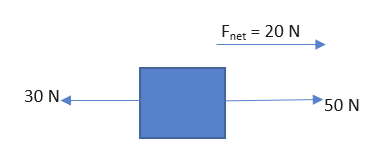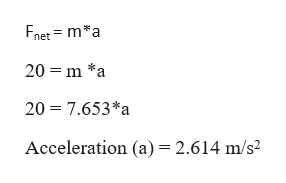An object that weighs 75 N is pulled on a horizontal surface by a horizontal pull of 50 N to the right. The friction force on this object is 30 N to the left. What is the acceleration of the object?

Question

An object that weighs 75 N is pulled on a horizontal surface by a horizontal pull of 50 N to the right. The friction force on this object is 30 N to the left. What is the acceleration of the object?

Step 1

Given weight of the object =75 N

Weight = mg =75

Mass of the object = 75/g = 75/9.8  = 7.653 kg.

Pulling force is acting to the right and it is 50 N

Frictional force is acting to the left of magnitude 30 N.

Net force acting on the object is = 50N-30N = 20 N

Net force is 20 N and is acting to right as shown in the figure.help_outlineImage TranscriptioncloseFnet 20 N 30 N 50 N fullscreen
Step 2

From Newton’s second l...help_outlineImage TranscriptioncloseFnet ma 20 m *a 20 7.653*a Acceleration (a) 2.614 m/s2 fullscreen

Want to see the full answer?

See Solution

Want to see this answer and more?

Our solutions are written by experts, many with advanced degrees, and available 24/7

See Solution
Tagged in

Newtons Laws of Motion